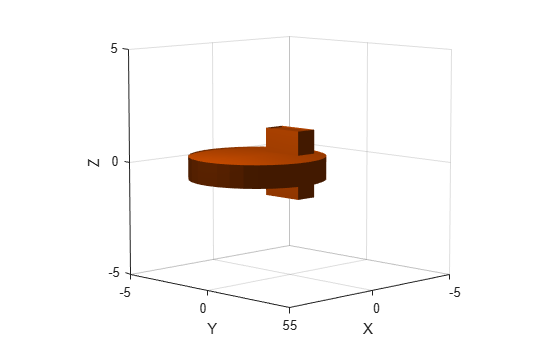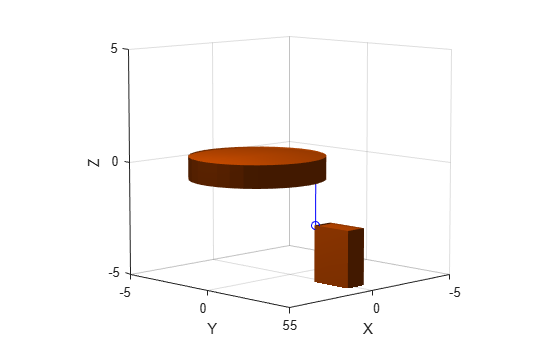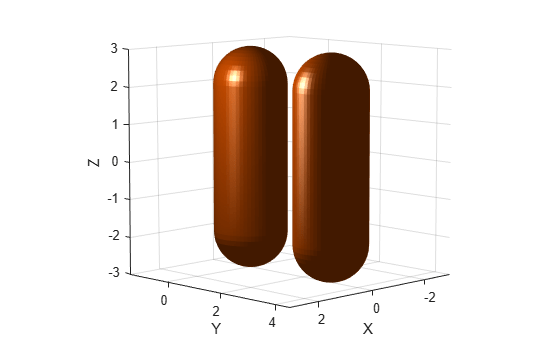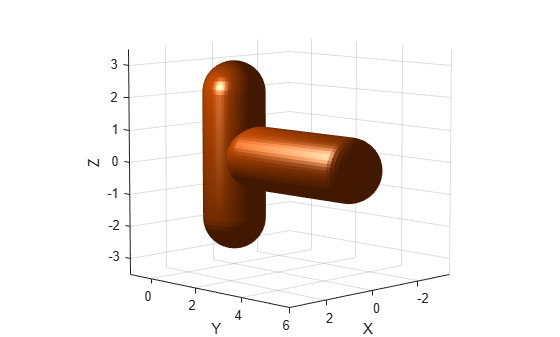# checkCollision

Check if two geometries are in collision

Since R2019b

## Syntax

``collisionStatus = checkCollision(geom1,geom2)``
``[collisionStatus,sepdist,witnesspts] = checkCollision(geom1,geom2)``

## Description

````collisionStatus = checkCollision(geom1,geom2)` returns the collision status between the two convex geometries `geom1` and `geom2`. If the two geometries are in collision at their specified poses, `collisionStatus` is `1`. If the function does not find a collision, `collisionStatus` is `0`.```

example

````[collisionStatus,sepdist,witnesspts] = checkCollision(geom1,geom2)` returns the minimal distance `sepdist` and witness points `witnesspts` of each geometry when the function does not find a collision between the two geometries.```

## Examples

collapse all

This example shows how to check the collision status of two collision geometries.

Create a box collision geometry.

`bx = collisionBox(1,2,3);`

Create a cylinder collision geometry.

`cy = collisionCylinder(3,1);`

Translate the cylinder along the x-axis by 2.

```T = trvec2tform([2 0 0]); cy.Pose = T;```

Plot the two geometries.

```show(cy) hold on show(bx) xlim([-5 5]) ylim([-5 5]) zlim([-5 5]) hold off```Check the collision status. Confirm the status is consistent with the plot.

`[areIntersecting,dist,witnessPoints] = checkCollision(bx,cy)`
```areIntersecting = 1 ```
```dist = NaN ```
```witnessPoints = 3×2 NaN NaN NaN NaN NaN NaN ```

Translate the box along the x-axis by 3 and down the z-axis by 4. Confirm the box and cylinder are not colliding.

```T = trvec2tform([0 3 -4]); bx.Pose = T; [areIntersecting,dist,witnessPoints] = checkCollision(bx,cy)```
```areIntersecting = 0 ```
```dist = 2 ```
```witnessPoints = 3×2 0.4286 0.4286 2.0000 2.0000 -2.5000 -0.5000 ```

Plot the box, cylinder, and the line segment representing the minimum distance between the two geometries.

```show(cy) hold on show(bx) wp = witnessPoints; plot3([wp(1,1) wp(1,2)], [wp(2,1) wp(2,2)], [wp(3,1) wp(3,2)], 'bo-') xlim([-5 5]) ylim([-5 5]) zlim([-5 5]) hold off```Create two collision capsules. Center one at the origin, and set the pose of the other capsule to `3` meters away from the origin on the y-axis. Display the capsules.

```cc1 = collisionCapsule(1,4); cc2 = collisionCapsule(1,4); cc2.Pose = trvec2tform([0 3 0]); show(cc1); hold on show(cc2); axis auto hold off```Check for collision between the two collision capsules. Because they are not visually colliding, the function should return real-valued separation distances and witness points. Display the separation distances and witness points.

```[isColliding,separationDist,witnessPts] = checkCollision(cc1,cc2); disp("Separation Distance: " + num2str(separationDist))```
```Separation Distance: 1 ```
`disp("Capsule 1 Witness Point (X Y Z): " + num2str(witnessPts(:,1)'))`
```Capsule 1 Witness Point (X Y Z): 0 1 -2 ```
`disp("Capsule 2 Witness Point (X Y Z): " + num2str(witnessPts(:,2)'))`
```Capsule 2 Witness Point (X Y Z): 0 2 -2 ```

Rotate the second capsule `90` degrees on the z-axis.

```cc2.Pose(1:3,1:3) = eul2rotm([0 0 pi/2]); show(cc1); hold on show(cc2); axis auto```Check again for collision between the capsules. Because they are in collision, the function returns the separation distance and witness points as `NaN`.

```[isColliding,separationDist,witnessPts] = checkCollision(cc1,cc2); disp("Separation Distance: " + num2str(separationDist))```
```Separation Distance: NaN ```
`disp("Capsule 1 Witness Point (X Y Z): " + num2str(witnessPts(:,1)'))`
```Capsule 1 Witness Point (X Y Z): NaN NaN NaN ```
`disp("Capsule 2 Witness Point (X Y Z): " + num2str(witnessPts(:,2)'))`
```Capsule 2 Witness Point (X Y Z): NaN NaN NaN ```

## Input Arguments

collapse all

First collision geometry, specified as one of these collision geometry objects:

Collision geometry, specified as one of these collision geometry objects:

## Output Arguments

collapse all

Collision status, returned as `0` or `1`. If the two geometries are in collision, `collisionStatus` is `1`. Otherwise, the value is `0`.

Data Types: `double`

Minimal distance between the two collision geometries, returned as a real number. The line segment that connects the witness points `witnesspts` determines the minimal distance between the two geometries. When the two geometries are in collision, `sepdist` is `NaN`.

Data Types: `double`

Witness points on each geometry, returned as a 3-by-2 matrix. Each column is the location of the witness point on the corresponding geometry, `geom1` or `geom2`. The line segment that connects the two witness points has length `septdist`. When the two geometries are in collision, every element of `witnesspts` is `NaN`.

Data Types: `double`

## Limitations

• Collision checking results are unreliable when the minimal distance is below 10-5 m.

 Gilbert, E.G., D.W. Johnson, and S.S. Keerthi. "A fast procedure for computing the distance between complex objects in three-dimensional space." IEEE Journal on Robotics and Automation 4, no. 2 (April 1988): 193–203. https://doi.org/10.1109/56.2083.